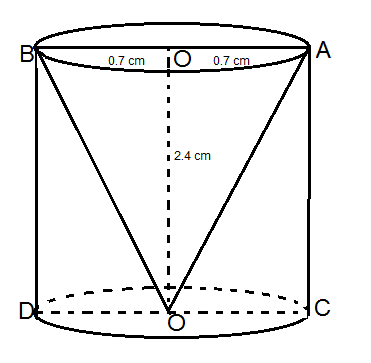From a solid cylinder whose height is 2.4cm and diameter 1.4cm, a conical cavity of the same height and the same diameter is hollowed out. The total surface area of the remaining solid to the nearest  c m 2  is ____.

# From a solid cylinder whose height is 2.4cm and diameter 1.4cm, a conical cavity of the same height and the same diameter is hollowed out. The total surface area of the remaining solid to the nearest   is ____.

Fill Out the Form for Expert Academic Guidance!l

+91

Live ClassesBooksTest SeriesSelf Learning

Verify OTP Code (required)

### Solution:According to the given information, a height of 2.4 cm of a solid cylinder with a diameter of 1.4 cm, where the same conical cavity is hollowed out at the same height and diameter
So the dimensions we know are
Height of full cylinder (v) = 2.4 cm
Diameter of solid cylinder (d) = 1.4 cm
Therefore, the radius of the solid cylinder (r) = 0.7 cm
As we know, the formula of the total surface of the remaining solid is equal to the curved surface of the cylinder + the area of ​​​​the base of the cylinder + the curved surface of the cone
Now we know that the curved surface of the cylinder =
Area of the base of the cylinder   and Curved surface area of the cone   .
Where   slant height which is given as
Therefore, Total surface area of the remaining solid =

Now substituting the values in the above formula, we get

So, Total surface area of the remaining solid is equal to  .

## Related content

 Area of Square Area of Isosceles Triangle Pythagoras Theorem Triangle Formula Perimeter of Triangle Formula Area Formulae Volume of Cone Formula Matrices and Determinants_mathematics Critical Points Solved Examples Type of relations_mathematics+91

Live ClassesBooksTest SeriesSelf Learning

Verify OTP Code (required)